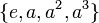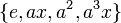# Non-inner non-simple fusion system for dihedral group:D8

Jump to: navigation, search
This article describes a particular fusion system on a group of prime power order, namely dihedral group:D8.
Get information on fusion systems for dihedral group:D8.
View other particular fusion systems

This article describes a fusion system on dihedral group:D8 that is neither the inner fusion system (the one induced purely by inner automorphisms of the group) nor the simple fusion system. It is an intermediate fusion system]].

Strictly speaking, there are two such fusion systems if we fix the group beforehand concretely. However, the two fusion systems are isomorphic as fusion systems. More explicitly, they are interchanged by any outer automorphism of dihedral group:D8.

## Explicit description

This fusion system is realized, for instance, in the symmetric group of degree four. In fact, the symmetric group of degree four is in essence the only example -- any example admits this as a subquotient. In the table below, we describe the subgroups explicitly using this realization, i.e., in terms of D8 in S4.

Equivalence class under isomorphisms, explicit description of subgroups Description of subgroups as permutation groups Subgroups involved Order Index Number of conjugacy classes of subgroups fused Total number of subgroups (=1 iff weakly closed subgroup for the fusion system) Are all group automorphisms of each subgroup included? Size of automorphism group from the fusion system Total number of isomorphisms (including automorphisms and others) = (number of automorphisms)$\times$ (number of subgroups)${}^2$
trivial subgroup$()$ 1 8 1 1 Yes 1 1$\{ e, a^2 \}, \{ e, ax \}, \{ e, a^3x \}$$\{ (), (1,3)(2,4)\}$,$\{ (), (1,4)(2,3) \}$,$\{ (), (1,2)(3,4) \}$ center of dihedral group:D8, non-normal subgroups of dihedral group:D8 (one of the two conjugacy classes) 2 4 2 3 Yes 1 9$\{ e, x \}, \{ e, a^2x \}$$\{ (), (1,3) \}, \{ (), (2,4) \}$ non-normal subgroups of dihedral group:D8 (one of the two conjugacy classes) 2 4 1 2 Yes 1 4$\{ e, a, a^2, a^3 \}$$\{ (), (1,2,3,4), (1,3)(2,4), (1,4,3,2) \}$ cyclic maximal subgroup of dihedral group:D8 4 2 1 1 Yes 2 2$\{ e, x, a^2, a^2x \}$$\{ (), (1,3), (1,3)(2,4), (2,4) \}$ Klein four-subgroups of dihedral group:D8 (one of them) 4 2 1 1 No (see below) 2 2$\{ e, ax, a^2, a^3x \}$$\{ (), (1,4)(2,3), (1,3)(2,4), (1,2)(3,4) \}$ Klein four-subgroups of dihedral group:D8 (one of them) 4 2 1 1 Yes 6 6
whole group 8 1 1 1 No (see below) 4 4
Total -- -- -- -- 8 10 -- -- --

Here is a description of the automorphism group from the fusion system in the two cases that it is not the full automorphism group:

• For the group$\{ e, x, a^2, a^2x \}$, which is one of the Klein four-subgroups of dihedral group:D8, the automorphisms arising from the fusion system are the identity map and the automorphism that interchanges$(1,3)$ with$(2,4)$, while fixing$()$ and$(1,3)(2,4)$.
• For the whole group, the automorphisms arising from the fusion system are precisely the inner automorphisms. This is true for all fusion systems on dihedral group:D8 because the outer automorphism group of this group is a 2-group, so it has no 2'-automorphisms.

## Realization in groups

Here are some examples of a group having dihedral group:D8 as a 2-Sylow subgroup such that this is the fusion system induced:

Group Order Dihedral group:D8 as a subgroup in this group Comment
symmetric group:S4 24 D8 in S4 this is the canonical, minimal example
symmetric group:S5 120 D8 in S5 the fusion system for this is precisely the same as that for D8 in S4 viewing symmetric group:S4 as an intermediate subgroup.